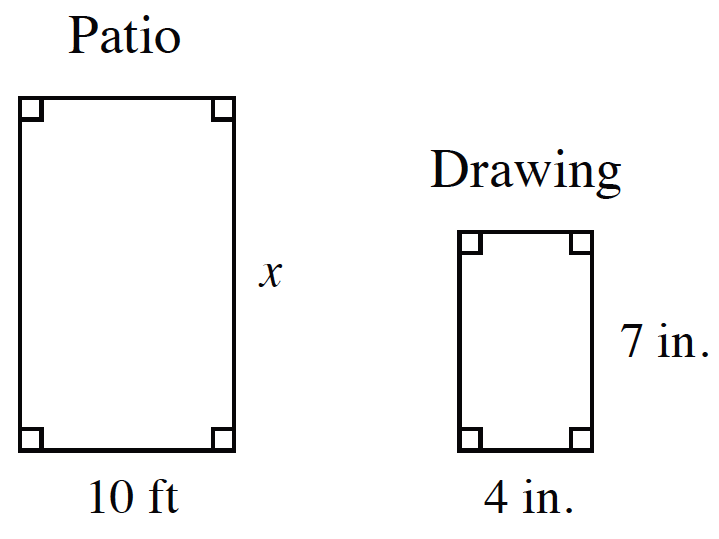### Home > ACC7 > Chapter 9 Unit 10 > Lesson CC3: 9.2.3 > Problem9-94

9-94.

Daniel needed to paint his patio, so he made a scale drawing of it. He knows that the width of the patio is $10$ feet, but the scale drawing is in inches.

1. Find the length of the patio in feet.

Find the ratio between two corresponding sides and use it to create a proportion or to multiply as a scale factor and find the missing length.

$x = 17.5$ feet

2. Find the area of the patio so Daniel knows how much area he needs to paint.

Use the answer that you got for part (a) to find the area of the patio.

Patio Area: $175$ square feet

3. One can of paint covers $125$ square feet. How many cans of paint will Daniel need to buy?

If each can of paint covers $125$ square feet, how many cans are needed to cover $175$ square feet?

Daniel is only able to buy full cans of paint. Round up the cans of paint if it does not come out to a whole number.